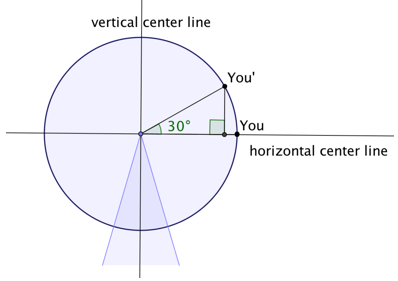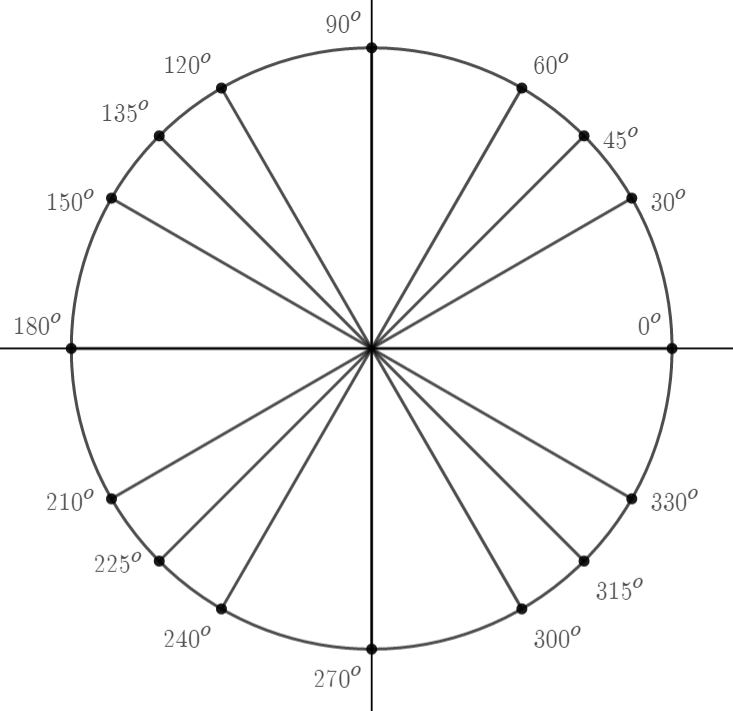# Unit Circle and Trigonometric Functions

## Objective

Evaluate sines and cosines of points at reference angles on the unit circle.

## Common Core Standards

### Core Standards

?

• F.TF.A.2 — Explain how the unit circle in the coordinate plane enables the extension of trigonometric functions to all real numbers, interpreted as radian measures of angles traversed counterclockwise around the unit circle.

• F.TF.A.4 — Use the unit circle to explain symmetry (odd and even) and periodicity of trigonometric functions.

?

• G.SRT.D.10

• G.SRT.D.11

• G.SRT.D.9

## Criteria for Success

?

• Describe geometrically why ${\mathrm{cos}\theta }$ represents the $x$-coordinate and ${\mathrm{sin}\theta }$ represents the $y$-coordinate on the unit circle.
• Use reference angles to evaluate sines and cosines on the unit circle.
• Define standard position, terminal side, and initial side of angles.
• Use correct notation for sines and cosines of angles.
• Identify the difference between positive and negative angles on the unit circle.

?

## Anchor Problems

?

### Problem 1

Below is a diagram of a Ferris wheel. It is a model with a one-foot radius.Assume that a person enters onto the Ferris wheel at the position You and travels a turn of ${30^{\circ}}$ round the center point.

1. How far above the horizontal center line is You' ?
2. How far away from the vertical center line is You' ?

### Problem 2

What are the sine and cosine for each degree turn shown below?### Problem 3

In the unit circles we have been looking at, ${0^{\circ}}$ and $36{0^{\circ}}$ are marked in the same place. What other angles are equivalent to $3{0^{\circ}}$$7{0^{\circ}}$? What angle on the unit circle is equivalent to ${495^{\circ}}$?

## Problem Set

?

The following resources include problems and activities aligned to the objective of the lesson that can be used to create your own problem set.

• Have students generate the unit circle without the use of their notes.
• Error analysis for:
• Reversing sine and cosine in the coordinate points
• Incorrect signs
• Without a calculator, find the sine and cosine for angles that are not in Quadrant I and are multiple turns around the circle.
• EngageNY Mathematics Algebra II > Module 2 > Topic A > Lesson 5Problem Set (Feel free to put in the work about right triangles. It will help later on, but it is not necessary for the objective of this lesson.)
• EngageNY Mathematics Algebra II > Module 2 > Topic A > Lesson 4Problem Set (Feel free to put in the work about right triangles. It will help later on, but it is not necessary for the objective of this lesson.)

?

### Problem 1

Calculate ${\mathrm{sin}(210^{\circ})}$ and ${\mathrm{cos}(210^{\circ})}$ without a calculator.

#### References

EngageNY Mathematics Algebra II > Module 2 > Topic A > Lesson 4Exit Ticket, Question #1

Algebra II > Module 2 > Topic A > Lesson 4 of the New York State Common Core Mathematics Curriculum from EngageNY and Great Minds. © 2015 Great Minds. Licensed by EngageNY of the New York State Education Department under the CC BY-NC-SA 3.0 US license. Accessed Dec. 2, 2016, 5:15 p.m..

Modified by The Match Foundation, Inc.

### Problem 2

Evaluate ${\mathrm{sin}(-90^{\circ})}$ without a calculator.

### Problem 3

Calculate ${\mathrm{cos}(480^{\circ})}$ and ${\mathrm{sin}(480^{\circ})}$ without a calculator.

#### References

EngageNY Mathematics Algebra II > Module 2 > Topic A > Lesson 5Exit Ticket, Question #1

Algebra II > Module 2 > Topic A > Lesson 5 of the New York State Common Core Mathematics Curriculum from EngageNY and Great Minds. © 2015 Great Minds. Licensed by EngageNY of the New York State Education Department under the CC BY-NC-SA 3.0 US license. Accessed Dec. 2, 2016, 5:15 p.m..

Modified by The Match Foundation, Inc.